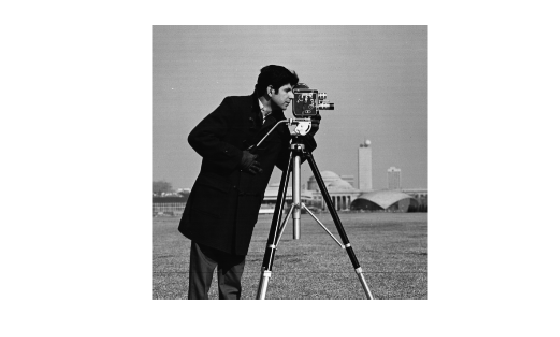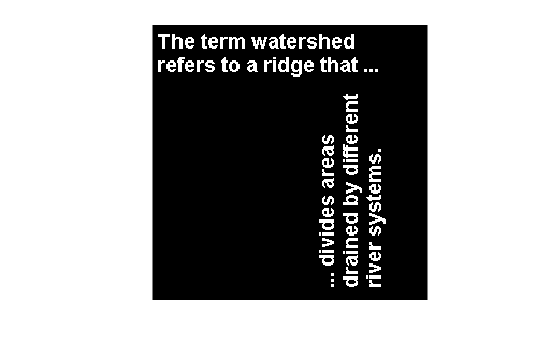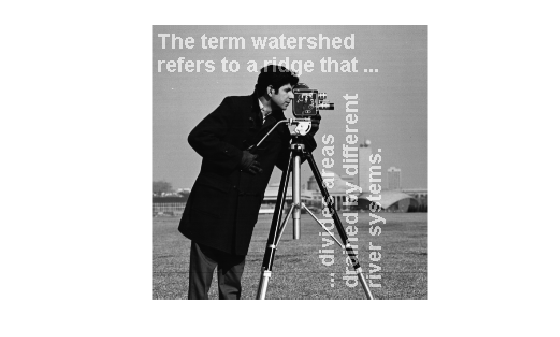# Apply Custom Filter to Region of Interest in Image

This example shows how to filter a region of interest (ROI), using the `roifilt2` function to specify the filter. `roifilt2` enables you to specify your own function to operate on the ROI. This example uses the `imadjust` function to lighten parts of an image.

Read an image into the workspace and display it.

```I = imread('cameraman.tif'); figure imshow(I)```Create the mask image. This example uses a binary image of text as the mask image. All the 1-valued pixels define the regions of interest. The example crops the image because a mask image must be the same size as the image to be filtered.

```BW = imread('text.png'); mask = BW(1:256,1:256); figure imshow(mask)```Create the function you want to use as a filter.

`f = @(x) imadjust(x,[],[],0.3);`

Filter the ROI, specifying the image to be filtered, the mask that defines the ROI, and the filter that you want to use.

`I2 = roifilt2(I,mask,f);`

Display the result.

```figure imshow(I2)```